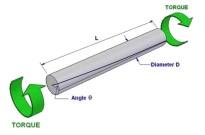# Question Help Pleaseeee .. .. .. .. For the part indicated. A) What would be the maximum torque that can be applied to the piece indicated if the bar is solid, made of steel, with a diameter of 5 cm, L=2m and the angle of total twist in the length cannot exceed 2 degrees? Assume G=80 Gpa J= pi*d^4/32 TORQUE - Dia TORQUEEKIIP8 The Asker · Mechanical Engineering

..

..

..

..

For the part indicated. A) What would be the maximum torque that can be applied to
the piece indicated if the bar is solid, made of steel, with a diameter of 5 cm, L=2m and the angle of
total twist in the length cannot exceed 2 degrees? Assume G=80 Gpa

J= pi*d^4/32Transcribed Image Text: TORQUE - Dia TORQUE
More
Transcribed Image Text: TORQUE - Dia TORQUE